XLL+ Class Library (7.0)

# Grouping multiple arguments into a single Excel range

Excel versions before 2007 only accept a limited number of arguments - 30. Therefore, you may sometimes find it convenient to group multiple arguments into a single input range. For instance, if your function accepts 35 arguments, you can group 6 related arguments together and require that they be passed in a single range of size 1 x 6 or 6 x 1.

The Function Wizard lets you specify grouped arguments, and generates code to read them from Excel ranges into local variables.

This walkthrough demonstrates how to create an add-in function that accepts grouped arguments.

Our add-in function will combine Excel's NORMDIST and NORMSDIST built-in functions, by accepting a value, and optionally a range containing a mean and standard deviation. If the range is omitted, then defaults of 0 and 1 will be used for the mean and the standard deviation respectively.

In a real-world example, a function would have dozens of other arguments. Grouping arguments when there is no need to is confusing and irritating for the user. We only do it here for the sake of a simpler example.

This walkthrough also covers two other areas:

• Optional arguments (with default values).
• Calling built-in Excel functions.

You can find a copy of all the code used in this walkthrough in the `Walkthroughs/GroupedArgs` folder in your XLL+ installation, along with a demonstration spreadsheet, `GroupedArgs.xls`.

### Creating the project

#### To create the project using Visual Studio

1. From the File menu, select New and then Project to open the New Project dialog.
2. Select the XLL+ 7 Excel Add-in project template from the list of Visual C++ Projects, and enter GroupedArgs in the Name box. Under Location, enter an appropriate directory in which to create the project.
3. Accept all the default settings in the XLL+ AppWizard.

For more information about creating projects, see Creating an add-in project in the XLL+ User Guide.

### Creating the function

Note: If you do not know how to start the Function Wizard, or you cannot find the command to open it, look at Invoke the Function Wizard in the User Guide.

1. If you are not already editing `GroupedArgs.cpp`, open it, using the Visual Studio Solution Explorer.

2. Use the XLL+ Function Wizard to add a new function, GroupedFn, filling in the function's name as shown below: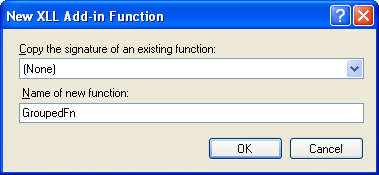Name GroupedFn CXlOper Statistical Function with grouped arguments
3. Fill in the function's category and description.

Add three numeric arguments, as shown below: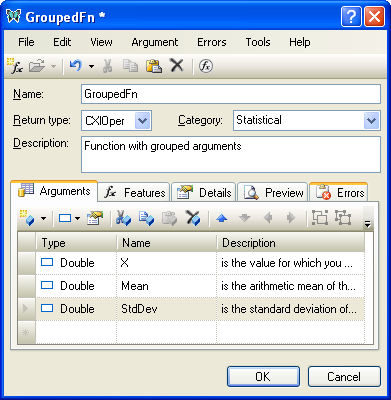Type Name Description
Double X is the value for which you want the distribution
Double Mean is the arithmetic mean of the distribution
Double StdDev is the standard deviation of the distribution
4. Select the row containing Mean, and click on the Argument details button (shown below) or press the `Alt+Enter` keys.5. In the Argument Details window, set Mean to be optional, and to have a default value of `0.0`, and click "OK".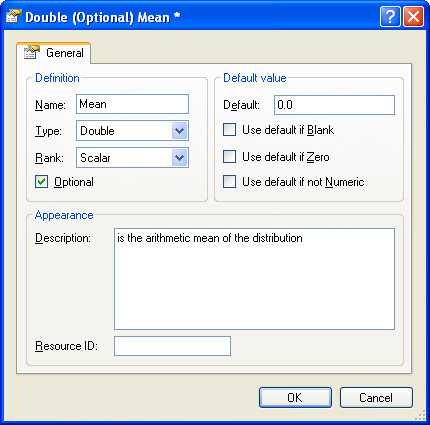6. Repeat the process with the third argument, StdDev, but with a default value of `1.0`.

The arguments should now appear as follows:

Type Name Description
Double X is the value for which you want the distribution
Double (=0.0) Mean is the arithmetic mean of the distribution
Double (=1.0) StdDev is the standard deviation of the distribution
7. Select the second argument, Mean, and press `Shift + Down-arrow` to select both the second and third arguments.8. Click on the Group tool-button (shown in red below).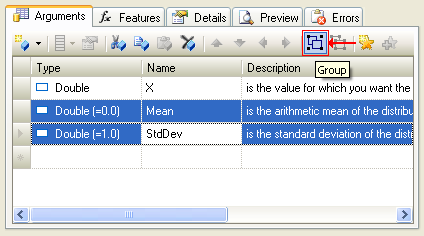9. A new argument Group1 has been added to the function, and Mean and StdDev are both within the group.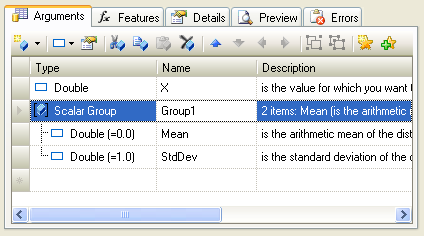10. Change the name of the group to be Distribution.

11. Scroll right until the Description column is fully visible, and inspect the list of descriptions that drop down when you click on the down-arrow.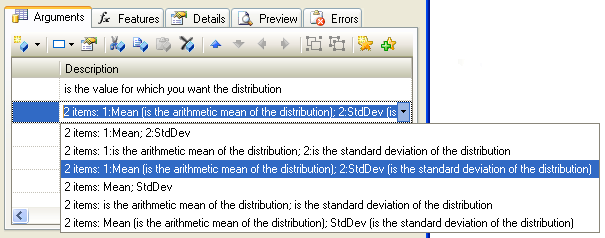Select the style which best fits the group. If you add new items to the group later, or reorder or edit the existing items, the description will automatically be updated to include the changes.

12. Click OK to accept the new function and close the Wizard.

### Examining the code

1. The Wizard has added code to GroupedArgs.cpp, as shown below.CopyC++
```CXlOper* GroupedFn_Impl(CXlOper& xloResult, double X, const CXlOper*
Distribution)
{
// Input buffers
double Mean;
double StdDev;
// Validate and translate inputs
static CScalarConvertParams<double> Mean__params(L"Mean", XLA_DEFAULT_EMPTY|
XLA_OPER_IS_GROUP, L"Distribution", 0, (double)0.0);
static CScalarConvertParams<double> StdDev__params(L"StdDev",
XLA_DEFAULT_EMPTY|XLA_OPER_IS_GROUP, L"Distribution", 1, (double)1.0);
// End of generated code
//}}XLP_SRC
// TODO - set the value of xloResult, or return another value
//          using CXlOper::RetXXX() or throw a CXlRuntimeException.
return xloResult.Ret();
}```
2. Examine the lines of code that extract the value Mean from the grouped argument Distribution:CopyC++
```double Mean;
static CScalarConvertParams<double> Mean__params(L"Mean", XLA_DEFAULT_EMPTY|
XLA_OPER_IS_GROUP, L"Distribution", 0, (double)0.0);

XlReadScalar() is used to extract Mean from the Excel argument Distribution. The two parameters `0, L"Mean"` (passed to XlReadScalar in the CScalarConvertParams object) are used to search the Excel array input named Distribution, by order and by name. The flag value `XLA_OPER_IS_GROUP` instructs XlReadScalar that the argument is a member of a group.

If the item cannot be found, or is empty, then the default value, `0.0`, is used. If the item is found, but cannot be converted to a number, then an exception of type CXlConversionException will be thrown, and input validation will stop immediately.

### Adding the code

1. Add some code to the add-in function to delegate all the work to the Excel built-in function NORMDIST, as shown below:CopyC++
```CXlOper* GroupedFn_Impl(CXlOper& xloResult, double X, const CXlOper*
Distribution)
{
// Input buffers
double Mean;
double StdDev;
// Validate and translate inputs
static CScalarConvertParams<double> Mean__params(L"Mean", XLA_DEFAULT_EMPTY|
XLA_OPER_IS_GROUP, L"Distribution", 0, (double)0.0);
static CScalarConvertParams<double> StdDev__params(L"StdDev",
XLA_DEFAULT_EMPTY|XLA_OPER_IS_GROUP, L"Distribution", 1, (double)1.0);
// End of generated code
//}}XLP_SRC

// xlfNormdist was looked up in the following XLL+ help topic:
// Section: Technical Notes
// Topic  : Excel built-in function numbers (Alphabetical order)
static int xlfNormdist = 293;

// Call Excel's built-in function NORMDIST
xloResult.Excel(xlfNormdist, 4, &CXlOper(X), &CXlOper(Mean),
&CXlOper(StdDev), &CXlOper(FALSE));

// Return results of call to NORMDIST
return xloResult.Ret();
}```
2. Note that for clarity, the function number of the built-in Excel functions is declared as a named static value: static int xlfNormdist = 293; This number was found by looking up the function in the table Excel built-in function numbers.

### Testing the add-in

1. Build the project, using the Build/Build Solution menu or Shift+Ctrl+B keyboard short-cut. If you are working with a 64-bit version of Excel, then be sure to select the 64-bit platform `x64` before you build.
2. When the project has been built successfully, use the Debug/Start Debugging menu or F5 keyboard short-cut to run your add-in under Excel.
3. If Excel puts up any message boxes asking whether you trust the add-in, say yes.
4. Set a break-point in your source code on the following line.CopyC++
```xloResult.Excel(xlfNormdist, 4, &CXlOper(X), &CXlOper(Mean),
&CXlOper(StdDev), &CXlOper(FALSE));```
5. In a spreadsheet cell, enter the following formula:
`=GroupedFn(2.3)`
6. Observe that the values of the local variables have been set to their default values.
7. In a spreadsheet cell, enter the following formula:
`=GroupedFn(2.3,{1,2})`
8. Observe that the values of the local variables Mean and StdDev have been set to 1.0 and 2.0 respectively.
9. In a spreadsheet cell, enter the following formula:
`=GroupedFn(2.3, {1, "Fred"})`
Note that an informative error message is returned:
`#ERROR: Expected number for Distribution (StdDev)`

### Extracting the values

In the formulae above, the Distribution argument was entered directly, as a pair of values, e.g. `{1,2}`. Normally, users would enter the argument as a range of cells. The function `XlReadScalar` does the work of unwrapping the values from the array argument.

#### Vector form

The grouped argument `Distribution` can be a vector containing the required elements in order, e.g.: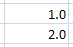or#### Labelled form

Alternatively, the grouped argument can contain two columns or rows, with labels in one and matching values in the other: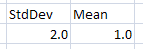Note that if labels are used the values can be in any order.

### Interactive help

If you build the interactive help for the project, you will see that the following help is available to the user if they click on "Help on this function" in the Excel Formula Wizard.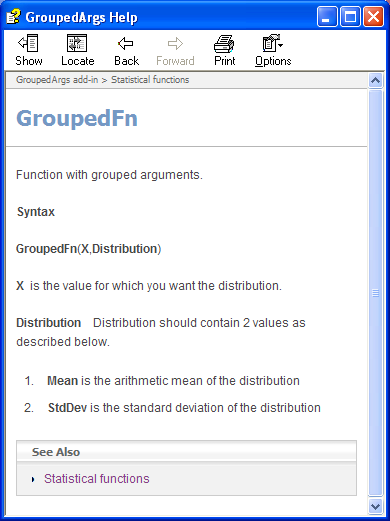This sort of help is essential for grouped arguments, which require extra care during input. See Help in the User guide for information on how to generate interactive help for an add-in.